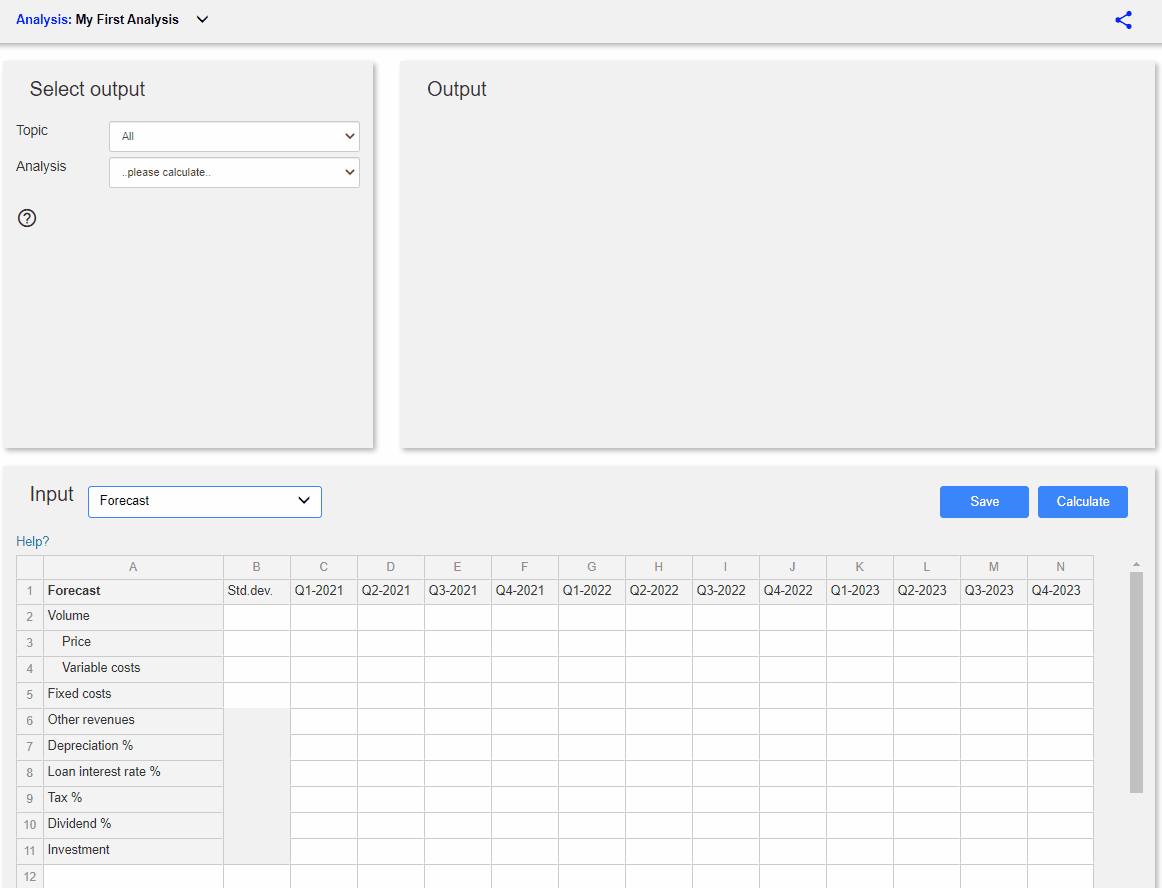## Financial Planning

Share and collaborate with others - Improve your decision making!

Financial Planning
Input expected sales, costs, investments etc.
Test different forecast assumptions.

• Income statements
• Balance sheets
• Cash flows
• Financial ratios
Equity Valuation
Create a financial forecast.
Select valution assumptions.

• Test different valuation methods and assumptions
• Include uncertainty and get the Equity valuation as a range estimate
• Impairment testing
Debt and Credit Analysis
Create a financial forecast.
Select debt/credit assumptions.

• Financing needs
• Debt capacity
• Credit rating & credit spread
• Probability of breaching debt covenants

## Financial Planning & Risk Management

Easily create a thorough and informative Financial Plan for your company

The Corporate Model - The complete Financial Planning and Risk Management Model

Financial projections
• Income statements, balance sheets, cash flows and ratios
• Consolidated, parent company and the subsidiaries

Debt management
• Debt need, debt capacity, Measure probability of breaching covenants
• Credit rating, credit spread and interest costs

Valuation and M&A analysis
• Valuation analysis and impairment testing
• Mergers & acquisitions analysis

Financial risk management and Derivatives
• Currency exposure - Interest rate exposure - Commodity exposure
• See how derivatives can change the exposures
• Find and communicate efficient hedging strategies
• Value at Risk (VaR), Cash Flow at Risk (CFaR), Profit at Risk (PaR)

## Financial Risk Management

Commodity exposure
Analyze and visualize price and volume risks.
Analyze the possible effect of hedging/derivatives.
Value at Risk (VaR), Cash Flow at Risk (CFaR), Profit at Risk (PaR)

NOREXECO

## Range forecasts

The models allow to include uncertainty assumptions* which then creates multipe forecasts presented as range forecasts.
* Standard deviations and correlations. These can be calculated automatically from the historical data.

#### Forecasts as fan charts

The black line shows the historical development.
The black dotted line shows the main case assumed future development.
The blue areas show the assumed possible future development with risk assumptions for the different confidence intervals.

#### Forecasts and estimations as range estimates

This example show the valuation as a range outcome.
The x-axis shows the distribution of calculated valuations/fair values.
The y-axis shows the calculated probabilities of each outcome.

# User-friendly### Analytical capabilities

Unparallelled analytical capabilities.
Allows to include uncertainty assumptions, and scenario testing.
Easy-to-understand visualization and communication of risk and opportunities.### Standardized

Calculations based on standard accounting rules, financial and statistical formulas.
Reduce modelling errors.You can upload your existing spreadsheet based forecasts into these solutions in order to access all the User-Friendliness our solutions provide.### Save time

Standardized models eliminates need for time consuming modelling setup.
Automatically updated reports.### Sharing and collaboration

Cloud/Web-based models allows for easy sharing and collaboration.
Easily select how, and if, you want to share and collaborate with other users.
It possible to collaborate live, copy sharing, publishing, full access or read only.### Extendable

Add analysis topics and features when and if it is needed.

### The Financial Planning Model